Test: Signal & Systems

# Test: Signal & Systems - Electrical Engineering (EE)

Test Description

## 25 Questions MCQ Test GATE Electrical Engineering (EE) 2024 Mock Test Series - Test: Signal & Systems

Test: Signal & Systems for Electrical Engineering (EE) 2023 is part of GATE Electrical Engineering (EE) 2024 Mock Test Series preparation. The Test: Signal & Systems questions and answers have been prepared according to the Electrical Engineering (EE) exam syllabus.The Test: Signal & Systems MCQs are made for Electrical Engineering (EE) 2023 Exam. Find important definitions, questions, notes, meanings, examples, exercises, MCQs and online tests for Test: Signal & Systems below.
Solutions of Test: Signal & Systems questions in English are available as part of our GATE Electrical Engineering (EE) 2024 Mock Test Series for Electrical Engineering (EE) & Test: Signal & Systems solutions in Hindi for GATE Electrical Engineering (EE) 2024 Mock Test Series course. Download more important topics, notes, lectures and mock test series for Electrical Engineering (EE) Exam by signing up for free. Attempt Test: Signal & Systems | 25 questions in 75 minutes | Mock test for Electrical Engineering (EE) preparation | Free important questions MCQ to study GATE Electrical Engineering (EE) 2024 Mock Test Series for Electrical Engineering (EE) Exam | Download free PDF with solutions
 1 Crore+ students have signed up on EduRev. Have you?
Test: Signal & Systems - Question 1

### The even part of the signal x(n) = u(n)

Detailed Solution for Test: Signal & Systems - Question 1

x(n) = u(n)
x(-n) = u(-n)
Even part of x(n),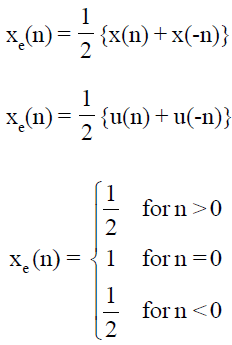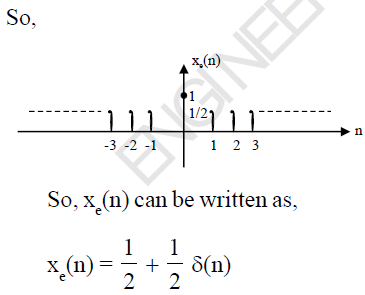Test: Signal & Systems - Question 2

### The value of discrete time signal at non-integer is :

Detailed Solution for Test: Signal & Systems - Question 2

The value of discrete time signal and non-integers is zero.

Test: Signal & Systems - Question 3

### The ROC of the signal x(t) = 4

Detailed Solution for Test: Signal & Systems - Question 3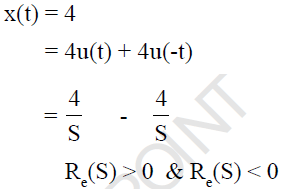Since there is no common ROC is ROC and laplace transform of x(t) does not exist.

Test: Signal & Systems - Question 4

The input and output relationship of a system is described as y(t) = ax + b, this system is linear

Detailed Solution for Test: Signal & Systems - Question 4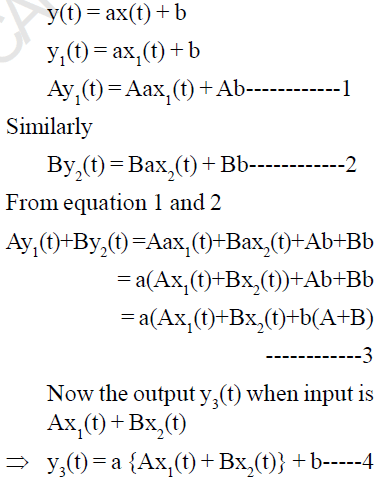For a linear system equation 3 and 4 must be equal, so b = 0, whereas for any value of a.

Test: Signal & Systems - Question 5

Which of the following signal is an example of an anti causal signal

Detailed Solution for Test: Signal & Systems - Question 5

For an anti causal signal, the value of a signal must be zero for t > 0 so signal is e-at u(-t)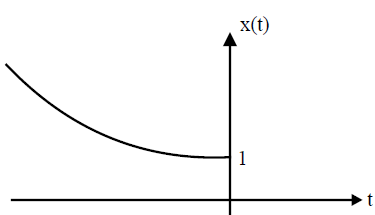Test: Signal & Systems - Question 6

The maximum phase-shift provided by the given system is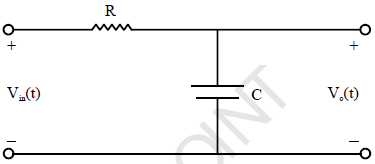Detailed Solution for Test: Signal & Systems - Question 6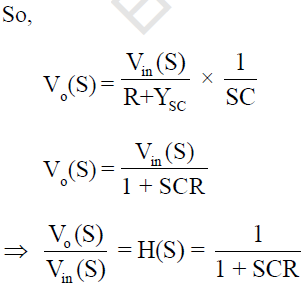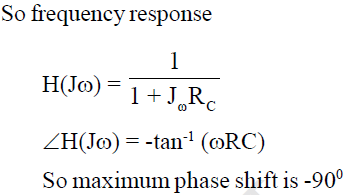Test: Signal & Systems - Question 7

Determine the fundamental period of x(n) =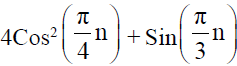Detailed Solution for Test: Signal & Systems - Question 7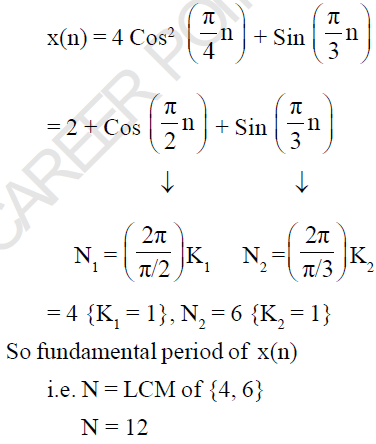Test: Signal & Systems - Question 8

Consider a signal x(t) = 4 rect (t/6) and its Fourier - transform is x(ω). Determine the area under the curve in the ω-domain.

Detailed Solution for Test: Signal & Systems - Question 8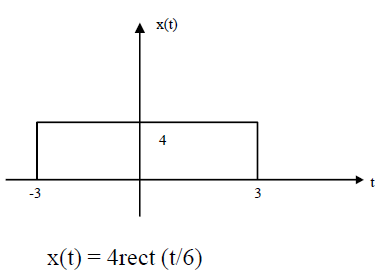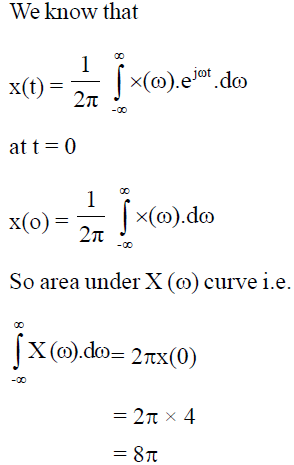Test: Signal & Systems - Question 9

If the Fourier transform of x(t) is x(ω), then determine the Fourier transform of x(at-b)

Detailed Solution for Test: Signal & Systems - Question 9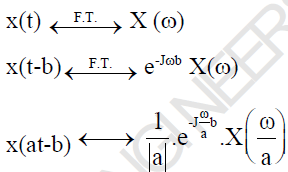Test: Signal & Systems - Question 10

A pole zero pattern of a certain filter is shown in the figure. The filter must be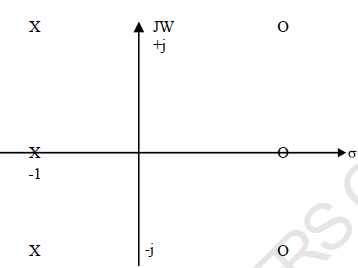Detailed Solution for Test: Signal & Systems - Question 10

Since locations of poles and zeros are mirror image to each other about vertical axis, so it is an all pass filter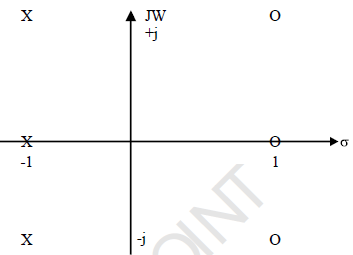Test: Signal & Systems - Question 11

Determine the laplace-transform of signal x(t) shown in figure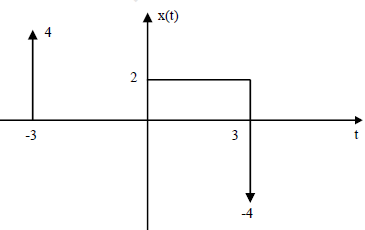Detailed Solution for Test: Signal & Systems - Question 11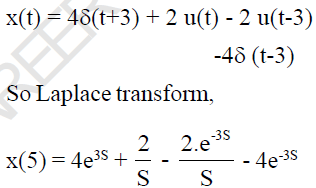Test: Signal & Systems - Question 12

Determine the total energy of x(t) = 12 Sin (6t)

Detailed Solution for Test: Signal & Systems - Question 12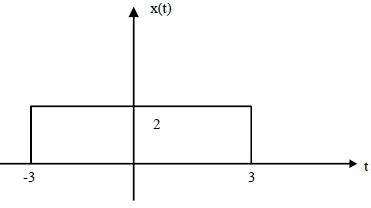x(t) = 12 Sinc (6t)
So, x(t) = 2 rect (f/6)
According to Parsvell's Theorem,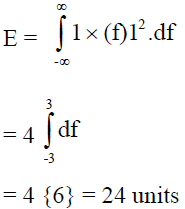Test: Signal & Systems - Question 13

Determine the bandwidth of the signal x(t) = e-at u(t), so that it contains 90% of its total energy.

Detailed Solution for Test: Signal & Systems - Question 13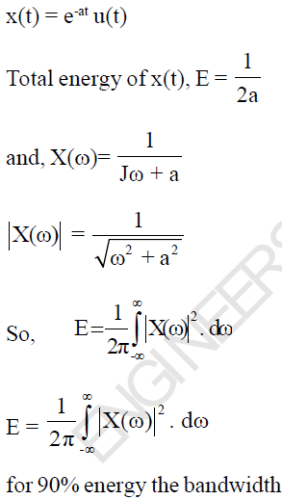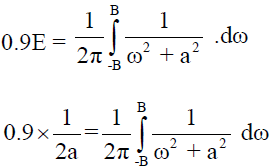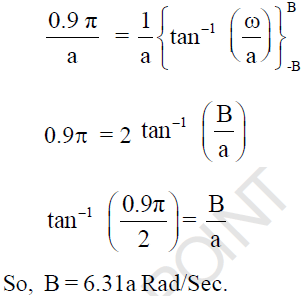Test: Signal & Systems - Question 14

Determine the output y(t) for an input x(t) = e-2t u(t), if the step response of the system is t u(t).

Detailed Solution for Test: Signal & Systems - Question 14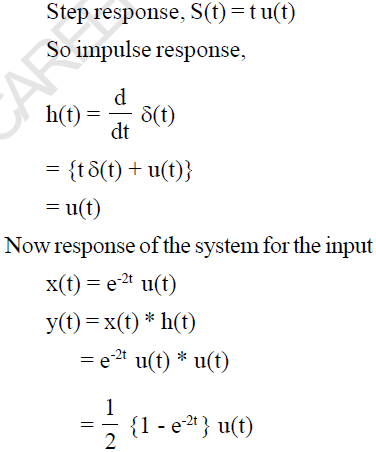Test: Signal & Systems - Question 15

Simplify the following expression:
δ(-t) * u(t) * e-t/2 u(t)

Detailed Solution for Test: Signal & Systems - Question 15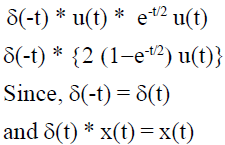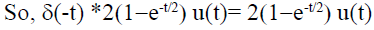Test: Signal & Systems - Question 16

Consider the analog signal x(t) = 3 Cos 100πt. This signal is sampled with a frequency of 75 samples per second and at the reconstruction side an ideal LPF having cut-off frequency is 30Hz is used. Determine  the frequency component at the output of filter.

Detailed Solution for Test: Signal & Systems - Question 16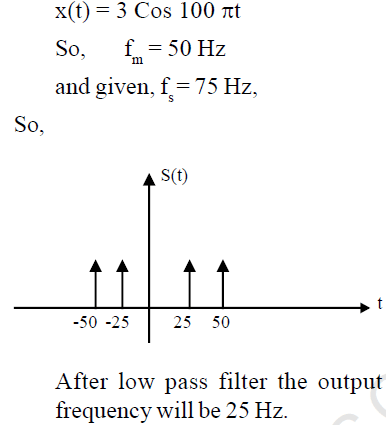Test: Signal & Systems - Question 17

The input and output relationship of a system is given as,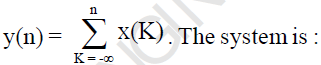Detailed Solution for Test: Signal & Systems - Question 17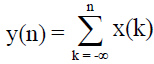It is the input-output relationship of an accumulator.
For any value of n, the output y(n) is depends only on the previous and present input
So it is causal.
It is also liner It is unstable system. Because the output is not bounded for the bounded input.

Test: Signal & Systems - Question 18

A signal x(t) = 2 (1 - Cos2πt) is sampled with a sampling frequency of 10 Hz. Determine the z-transform of sampled signal.

Detailed Solution for Test: Signal & Systems - Question 18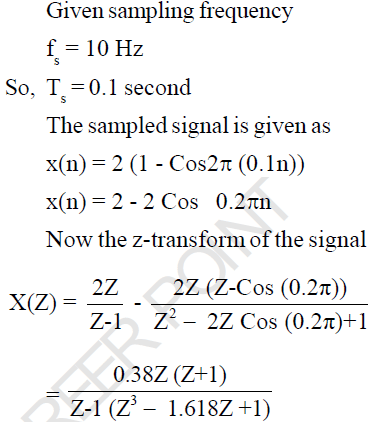Test: Signal & Systems - Question 19

Given,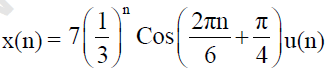Determine the ROC of its z-transform.

Detailed Solution for Test: Signal & Systems - Question 19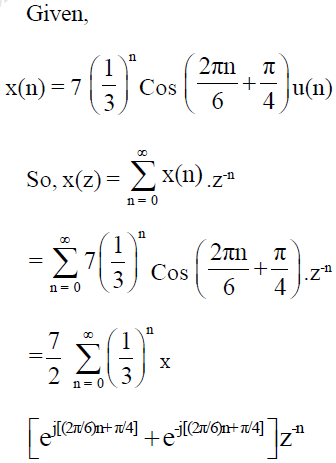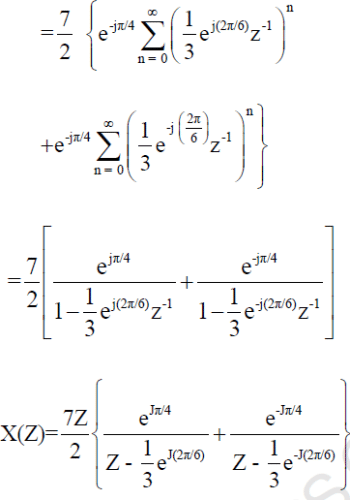ROC : |Z| > 1/3

Test: Signal & Systems - Question 20

If a signal f(t) has energy E, the energy of the signal f(2t) is equal to

Detailed Solution for Test: Signal & Systems - Question 20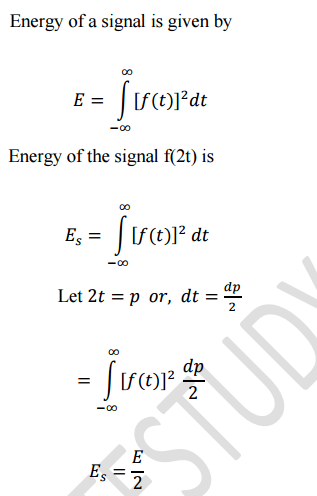Test: Signal & Systems - Question 21

The correlation between two signals x1(t) and x2(t) is 6. If average power of x1(t) is 10 and x2(t) is 8.
Then determine the power of x1(t) + x2(t)

Detailed Solution for Test: Signal & Systems - Question 21

Total energy is given as,
E = E1 + E2 + 2 Rx (τ)
= 10 + 8 + 2 × 6
= 30

Test: Signal & Systems - Question 22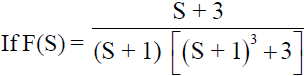, the co-efficient of term e-t in f(t) will be :

Detailed Solution for Test: Signal & Systems - Question 22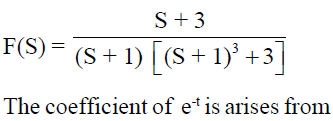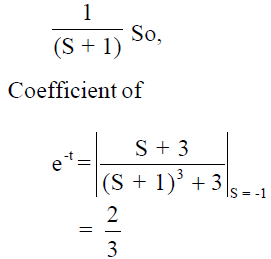Test: Signal & Systems - Question 23

S1 : δ(n) is an energy signal having energy is 1.

S2 : u(n) is a power signal having power is 1/2.

Which of the above is/are correct?

Detailed Solution for Test: Signal & Systems - Question 23

Energy of the signal is given as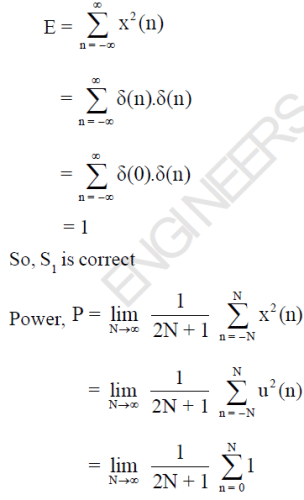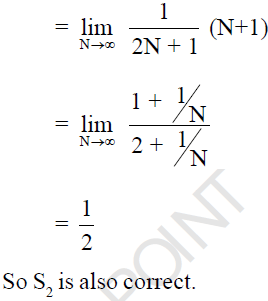Test: Signal & Systems - Question 24

The input and output relationship of a system is given as,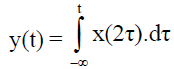The system is :

Detailed Solution for Test: Signal & Systems - Question 24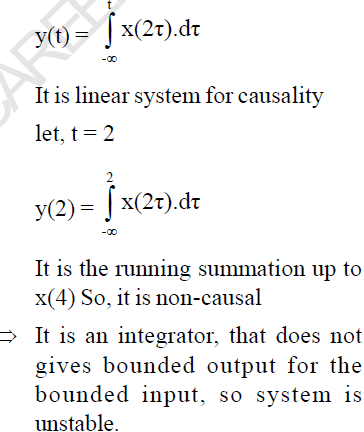Test: Signal & Systems - Question 25

Determine the impulse response of the inverse system, if the impulse response of the system is u(n).

Detailed Solution for Test: Signal & Systems - Question 25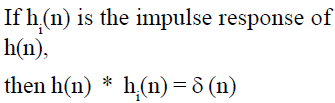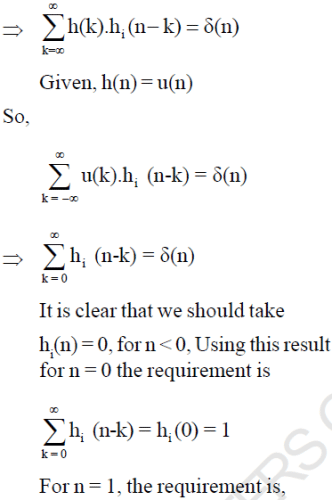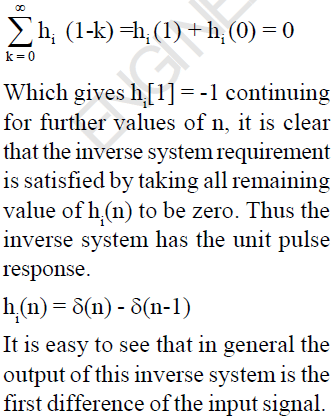## GATE Electrical Engineering (EE) 2024 Mock Test Series

23 docs|285 tests
Information about Test: Signal & Systems Page
In this test you can find the Exam questions for Test: Signal & Systems solved & explained in the simplest way possible. Besides giving Questions and answers for Test: Signal & Systems, EduRev gives you an ample number of Online tests for practice

## GATE Electrical Engineering (EE) 2024 Mock Test Series

23 docs|285 tests